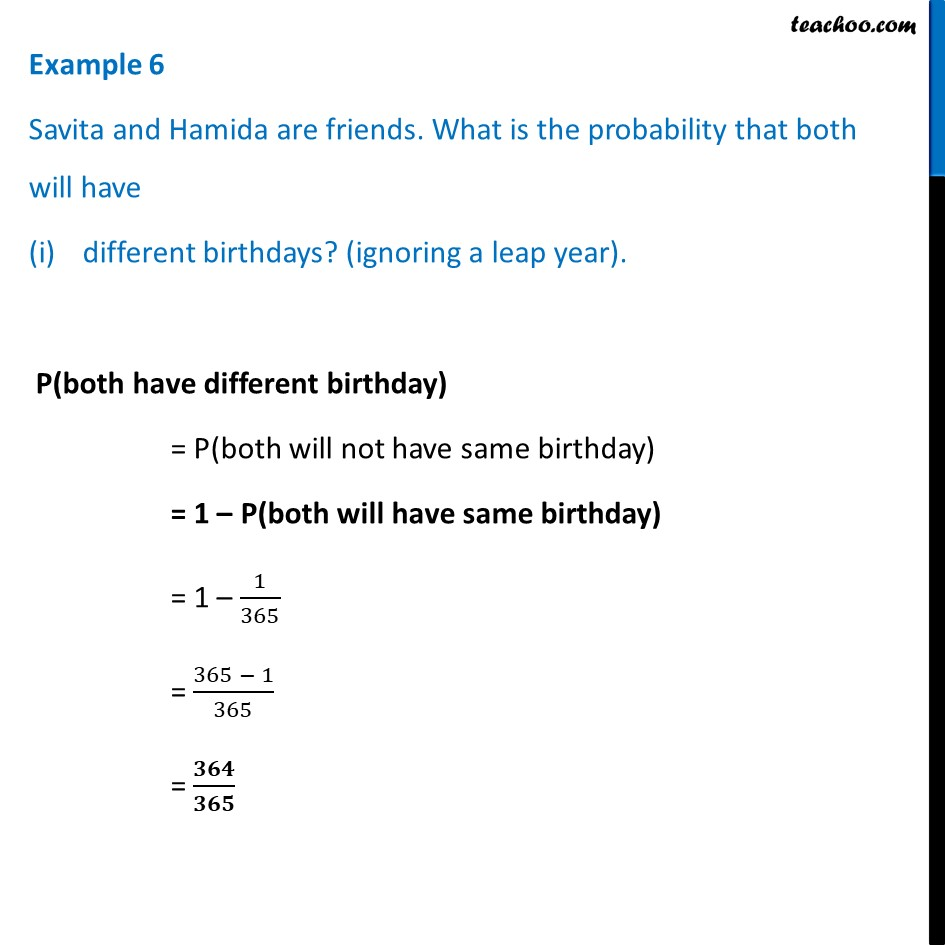Examples

Chapter 14 Class 10 Probability
Serial order wiseLearn in your speed, with individual attention - Teachoo Maths 1-on-1 Class

### Transcript

Example 6 Savita and Hamida are friends. What is the probability that both will have different birthdays? (ignoring a leap year). P(both have different birthday) = P(both will not have same birthday) = 1 – P(both will have same birthday) = 1 – 1/365 = (365 − 1)/365 = 𝟑𝟔𝟒/𝟑𝟔𝟓 Example 6 Savita and Hamida are friends. What is the probability that both will have (ii) same birthday? (ignoring a leap year). Number of days in an year = 365 Number of days when same birthday is possible = 1 P(both have same birthday) = (𝑁𝑢𝑚𝑏𝑒𝑟 𝑜𝑓 𝑑𝑎𝑦𝑠 𝑤ℎ𝑒𝑟𝑒 𝑡ℎ𝑒𝑦 𝑐𝑎𝑛 ℎ𝑎𝑣𝑒 𝑠𝑎𝑚𝑒 𝑏𝑖𝑟𝑡ℎ𝑑𝑎𝑦 𝑖𝑛 𝑎𝑛 𝑦𝑒𝑎𝑟)/(𝑇𝑜𝑡𝑎𝑙 𝑛𝑢𝑚𝑏𝑒𝑟 𝑜𝑓 𝑑𝑎𝑦𝑠 𝑖𝑛 𝑎𝑛 𝑦𝑒𝑎𝑟) = 𝟏/𝟑𝟔𝟓